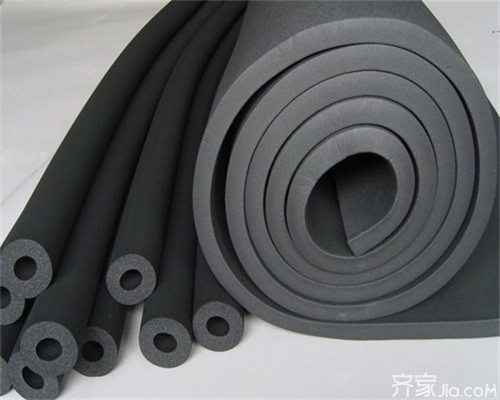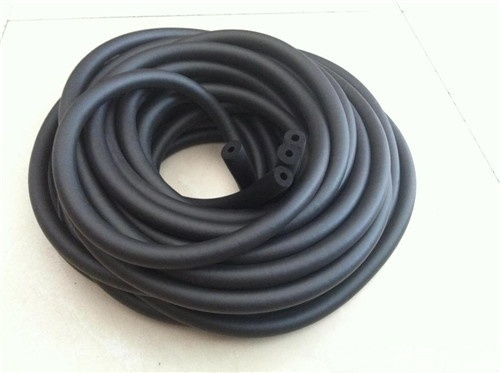|

# 橡塑管好不好 橡塑管生产厂家介绍

橡塑管好不好？橡塑管使用起来十分安全，既不会刺激皮肤，也不会危害健康。目前在各种工业中橡塑管被广泛的应用，不仅弹性好，而且，不吸水，不易燃烧，使用寿命长，对于工业的发展是大有帮助的。当然橡塑管在各种工业的使用越来越广泛了。那么，这种橡塑管生产厂家又有哪些呢？下面，就一起跟着小编去了解一下吧！橡塑管好不好：

1、绿色环保：不含有大气层有害的氯氟化物，符合ISO14000国际环保认证要求，所以在安装及应用中不会产生任何对人体有害的污染物。

2、导热系数低：橡塑是高品质的保温节能材料，是隔冷、隔热防结露的克星，热传导系数低并且保持稳定，对任何热介质起隔绝效果。

3、闭泡式结构：橡塑为闭泡式结构，外界空气中的水很难渗透到材料之中，具有优异的抗水汽渗透能力，保冷保温层外表不必再添加隔汽层。橡塑的湿阻因子μ值大于3500(ISO9346)构成内置的防水汽层，即使产品划伤也不影响整体的隔汽性。橡塑即是保温层又是防潮层。

4、用料薄、省空间橡塑使用厚度比其它保温材料减少三分之二左右。因而能节省楼层吊顶以上空间，提高室内高度。

5、使用寿命长：橡塑具有卓越的耐天候、抗老化、抗严寒、抗炎热、抗干燥、抗潮湿，还具有抗紫外线、耐臭氧、二十五年不老化、不变形、免维护使用寿命等特性。

6、防火性能好：橡塑材料符合国家标准GB8624《建筑材料燃烧性能分析法》经测试判定为GB8624B1级难燃性材料。

7、外观高档、匀整美观橡塑具有高弹性、平滑的表层，质地柔软，即使装在弯管、三通、阀门等不规则构件上都可以保持完整、美观，外表不须装饰，即使不吊顶也可保有高档性。

8、安装方便、快捷由于材质柔软，且无须其它辅助层，施工安装简易，对于管道的安装，可随管道安装的进度。橡塑管的特性

1、抗震性能：橡塑保温管具有很高的弹性，因而能最大限度地减少冷冻水和热水管道在使用过程中的振动和共振。

2、柔韧性能：橡塑保温管具有良好的绕性及韧性，施工中容易处理弯曲和不规则的管道，而且可以省工省料。

3、安装方便，外形美观：因本产品富柔软性，安装简易方便。管道安装：可套上后一起安装，也可将本管材纵向切开后再用铝箔胶带粘合而成。对阀门、三通、弯头等复杂部件，可将板材裁剪后，按不同形状包上粘合，确保整个系统的严密性，从而保证了整个系统的保温性。又因本材料外表有橡胶的光滑平整，以及它本身的优异性能，不需另加隔汽层，防护层，减少了施工中的麻烦，也保证了外形美观、平整。当设备或管道检修时，剥离下来的本材料可重复使用，性能不变。

4、其它优点：橡塑保温管使用起来十分安全，既不会刺激皮肤，也不会危害健康。它们能防止霉菌生长，避免害虫或老鼠啮咬，而且耐酸抗碱，性能优越。这些性能使华美橡塑成为保护管道的理想绝热材料，防止它们因大气介质或工业环境而受到腐蚀。橡塑管生产厂家

1、河北恒锦橡塑制品有限公司

公司简介：河北恒锦橡塑制品有限公司系ISO9001：2000质量管理体系认证企业，始建于1991年，技术力量雄厚，工艺设备先进，是生产高低压橡胶管，橡胶制品以及硅胶制品的生产厂家。

主营产品：高压油管、大口径胶管、液压油管、橡胶管、钢丝编织胶管、钢丝缠绕胶管、耐高温蒸汽胶管、耐磨喷砂胶管、高压耐磨胶管、夹布胶管、尼龙树脂管、耐油胶管等等。

2、江苏千富之丰科技有限公司

公司简介：江苏千富之丰科技有限公司BRAKETEC位于江苏省宜兴市经济技术开发区，是一家集特种橡胶开发设计、生产、销售，汽车制动软管生产与研发为一体的高新技术企业。

主营产品：汽车制动管、高温气动制动软管、氟橡胶条、氟橡胶管、高温气动制动软管总成、其它特种橡胶制品等等。

3、衡水海马橡塑有限公司

公司简介：衡水海马橡塑有限公司位于河北省东南部，京沪线、石德线、京福高速、济石高速、京济公路都由此经过，交通十分便利，地理位置十分优越，、生产销售橡塑制品为主的科研型企业。

主营产品：胶管、大口径法兰胶管、高压胶管、液压胶管、夹布胶管、金属软管、液压管件、PVC软管、各种车辆专用管、食品胶管、由壬等等。

编辑总结：关于橡塑管好不好、橡塑管生产厂家介绍的相关信息就为大家介绍到这里了，希望这篇文章对大家有所帮助。如果大家还有什么不明白的地方可以在下方给小编留言哦，我们会尽快为您解答。

`声明：本文由入驻焦点开放平台的作者撰写，除焦点官方账号外，观点仅代表作者本人，不代表焦点立场错误信息举报电话： 400-099-0099，邮箱：jubao@vip.sohu.com，或点此进行意见反馈，或点此进行举报投诉。`A B C D E F G H J K L M N P Q R S T W X Y Z
A - B - C - D - E
• A
• 鞍山
• 安庆
• 安阳
• 安顺
• 安康
• 澳门
• B
• 北京
• 保定
• 包头
• 巴彦淖尔
• 本溪
• 蚌埠
• 亳州
• 滨州
• 北海
• 百色
• 巴中
• 毕节
• 保山
• 宝鸡
• 白银
• 巴州
• C
• 承德
• 沧州
• 长治
• 赤峰
• 朝阳
• 长春
• 常州
• 滁州
• 池州
• 长沙
• 常德
• 郴州
• 潮州
• 崇左
• 重庆
• 成都
• 楚雄
• 昌都
• 慈溪
• 常熟
• D
• 大同
• 大连
• 丹东
• 大庆
• 东营
• 德州
• 东莞
• 德阳
• 达州
• 大理
• 德宏
• 定西
• 儋州
• 东平
• E
• 鄂尔多斯
• 鄂州
• 恩施
F - G - H - I - J
• F
• 抚顺
• 阜新
• 阜阳
• 福州
• 抚州
• 佛山
• 防城港
• G
• 赣州
• 广州
• 桂林
• 贵港
• 广元
• 广安
• 贵阳
• 固原
• H
• 邯郸
• 衡水
• 呼和浩特
• 呼伦贝尔
• 葫芦岛
• 哈尔滨
• 黑河
• 淮安
• 杭州
• 湖州
• 合肥
• 淮南
• 淮北
• 黄山
• 菏泽
• 鹤壁
• 黄石
• 黄冈
• 衡阳
• 怀化
• 惠州
• 河源
• 贺州
• 河池
• 海口
• 红河
• 汉中
• 海东
• I
• J
• 晋中
• 锦州
• 吉林
• 鸡西
• 佳木斯
• 嘉兴
• 金华
• 景德镇
• 九江
• 吉安
• 济南
• 济宁
• 焦作
• 荆门
• 荆州
• 江门
• 揭阳
• 金昌
• 酒泉
• 嘉峪关
K - L - M - N - P
• K
• 开封
• 昆明
• 昆山
• L
• 廊坊
• 临汾
• 辽阳
• 连云港
• 丽水
• 六安
• 龙岩
• 莱芜
• 临沂
• 聊城
• 洛阳
• 漯河
• 娄底
• 柳州
• 来宾
• 泸州
• 乐山
• 六盘水
• 丽江
• 临沧
• 拉萨
• 林芝
• 兰州
• 陇南
• M
• 牡丹江
• 马鞍山
• 茂名
• 梅州
• 绵阳
• 眉山
• N
• 南京
• 南通
• 宁波
• 南平
• 宁德
• 南昌
• 南阳
• 南宁
• 内江
• 南充
• P
• 盘锦
• 莆田
• 平顶山
• 濮阳
• 攀枝花
• 普洱
• 平凉
Q - R - S - T - W
• Q
• 秦皇岛
• 齐齐哈尔
• 衢州
• 泉州
• 青岛
• 清远
• 钦州
• 黔南
• 曲靖
• 庆阳
• R
• 日照
• 日喀则
• S
• 石家庄
• 沈阳
• 双鸭山
• 绥化
• 上海
• 苏州
• 宿迁
• 绍兴
• 宿州
• 三明
• 上饶
• 三门峡
• 商丘
• 十堰
• 随州
• 邵阳
• 韶关
• 深圳
• 汕头
• 汕尾
• 三亚
• 三沙
• 遂宁
• 山南
• 商洛
• 石嘴山
• T
• 天津
• 唐山
• 太原
• 通辽
• 铁岭
• 泰州
• 台州
• 铜陵
• 泰安
• 铜仁
• 铜川
• 天水
• 天门
• W
• 乌海
• 乌兰察布
• 无锡
• 温州
• 芜湖
• 潍坊
• 威海
• 武汉
• 梧州
• 渭南
• 武威
• 吴忠
• 乌鲁木齐
X - Y - Z
• X
• 邢台
• 徐州
• 宣城
• 厦门
• 新乡
• 许昌
• 信阳
• 襄阳
• 孝感
• 咸宁
• 湘潭
• 湘西
• 西双版纳
• 西安
• 咸阳
• 西宁
• 仙桃
• 西昌
• Y
• 运城
• 营口
• 盐城
• 扬州
• 鹰潭
• 宜春
• 烟台
• 宜昌
• 岳阳
• 益阳
• 永州
• 阳江
• 云浮
• 玉林
• 宜宾
• 雅安
• 玉溪
• 延安
• 榆林
• 银川
• Z
• 张家口
• 镇江
• 舟山
• 漳州
• 淄博
• 枣庄
• 郑州
• 周口
• 驻马店
• 株洲
• 张家界
• 珠海
• 湛江
• 肇庆
• 中山
• 自贡
• 资阳
• 遵义
• 昭通
• 张掖
• 中卫

1室1厅1厨1卫1阳台

1
2
3
4
5

0
1
2

1

1

0
1
2
3报名成功，资料已提交审核A B C D E F G H J K L M N P Q R S T W X Y Z
A - B - C - D - E
• A
• 鞍山
• 安庆
• 安阳
• 安顺
• 安康
• 澳门
• B
• 北京
• 保定
• 包头
• 巴彦淖尔
• 本溪
• 蚌埠
• 亳州
• 滨州
• 北海
• 百色
• 巴中
• 毕节
• 保山
• 宝鸡
• 白银
• 巴州
• C
• 承德
• 沧州
• 长治
• 赤峰
• 朝阳
• 长春
• 常州
• 滁州
• 池州
• 长沙
• 常德
• 郴州
• 潮州
• 崇左
• 重庆
• 成都
• 楚雄
• 昌都
• 慈溪
• 常熟
• D
• 大同
• 大连
• 丹东
• 大庆
• 东营
• 德州
• 东莞
• 德阳
• 达州
• 大理
• 德宏
• 定西
• 儋州
• 东平
• E
• 鄂尔多斯
• 鄂州
• 恩施
F - G - H - I - J
• F
• 抚顺
• 阜新
• 阜阳
• 福州
• 抚州
• 佛山
• 防城港
• G
• 赣州
• 广州
• 桂林
• 贵港
• 广元
• 广安
• 贵阳
• 固原
• H
• 邯郸
• 衡水
• 呼和浩特
• 呼伦贝尔
• 葫芦岛
• 哈尔滨
• 黑河
• 淮安
• 杭州
• 湖州
• 合肥
• 淮南
• 淮北
• 黄山
• 菏泽
• 鹤壁
• 黄石
• 黄冈
• 衡阳
• 怀化
• 惠州
• 河源
• 贺州
• 河池
• 海口
• 红河
• 汉中
• 海东
• I
• J
• 晋中
• 锦州
• 吉林
• 鸡西
• 佳木斯
• 嘉兴
• 金华
• 景德镇
• 九江
• 吉安
• 济南
• 济宁
• 焦作
• 荆门
• 荆州
• 江门
• 揭阳
• 金昌
• 酒泉
• 嘉峪关
K - L - M - N - P
• K
• 开封
• 昆明
• 昆山
• L
• 廊坊
• 临汾
• 辽阳
• 连云港
• 丽水
• 六安
• 龙岩
• 莱芜
• 临沂
• 聊城
• 洛阳
• 漯河
• 娄底
• 柳州
• 来宾
• 泸州
• 乐山
• 六盘水
• 丽江
• 临沧
• 拉萨
• 林芝
• 兰州
• 陇南
• M
• 牡丹江
• 马鞍山
• 茂名
• 梅州
• 绵阳
• 眉山
• N
• 南京
• 南通
• 宁波
• 南平
• 宁德
• 南昌
• 南阳
• 南宁
• 内江
• 南充
• P
• 盘锦
• 莆田
• 平顶山
• 濮阳
• 攀枝花
• 普洱
• 平凉
Q - R - S - T - W
• Q
• 秦皇岛
• 齐齐哈尔
• 衢州
• 泉州
• 青岛
• 清远
• 钦州
• 黔南
• 曲靖
• 庆阳
• R
• 日照
• 日喀则
• S
• 石家庄
• 沈阳
• 双鸭山
• 绥化
• 上海
• 苏州
• 宿迁
• 绍兴
• 宿州
• 三明
• 上饶
• 三门峡
• 商丘
• 十堰
• 随州
• 邵阳
• 韶关
• 深圳
• 汕头
• 汕尾
• 三亚
• 三沙
• 遂宁
• 山南
• 商洛
• 石嘴山
• T
• 天津
• 唐山
• 太原
• 通辽
• 铁岭
• 泰州
• 台州
• 铜陵
• 泰安
• 铜仁
• 铜川
• 天水
• 天门
• W
• 乌海
• 乌兰察布
• 无锡
• 温州
• 芜湖
• 潍坊
• 威海
• 武汉
• 梧州
• 渭南
• 武威
• 吴忠
• 乌鲁木齐
X - Y - Z
• X
• 邢台
• 徐州
• 宣城
• 厦门
• 新乡
• 许昌
• 信阳
• 襄阳
• 孝感
• 咸宁
• 湘潭
• 湘西
• 西双版纳
• 西安
• 咸阳
• 西宁
• 仙桃
• 西昌
• Y
• 运城
• 营口
• 盐城
• 扬州
• 鹰潭
• 宜春
• 烟台
• 宜昌
• 岳阳
• 益阳
• 永州
• 阳江
• 云浮
• 玉林
• 宜宾
• 雅安
• 玉溪
• 延安
• 榆林
• 银川
• Z
• 张家口
• 镇江
• 舟山
• 漳州
• 淄博
• 枣庄
• 郑州
• 周口
• 驻马店
• 株洲
• 张家界
• 珠海
• 湛江
• 肇庆
• 中山
• 自贡
• 资阳
• 遵义
• 昭通
• 张掖
• 中卫• 手机• 分享
• 设计
免费设计
• 计算器
装修计算器
• 入驻
合作入驻
• 联系
联系我们
• 置顶
返回顶部